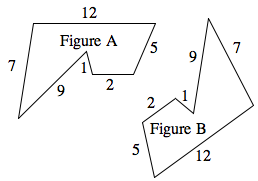### Home > CC2MN > Chapter 4 > Lesson 4.4.1 > Problem4-130

4-130.In the polygons at right, all corresponding angles are the same and the side lengths are as shown.

1. Find the ratios of the corresponding sides.

Since all corresponding angles are the same, the shape must be similar. Find the corresponding matching sides of the shape and compare the side lengths. What do you see?

The ratio is $1:1$

2. Are the two figures similar?

Similar shapes have the same angle measures and proportionate sides.

3. What is the special name for similar figures with a scale factor of $\frac { 1 } { 1 }$?

Congruent

4. What does this tell you about the side lengths and the angles of the two shapes?

Are the side lengths and angle measures for both shapes the same?